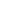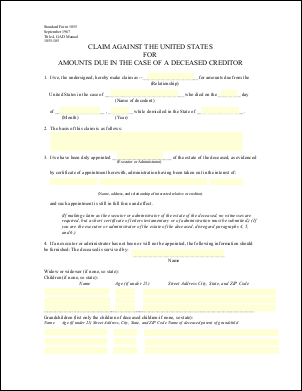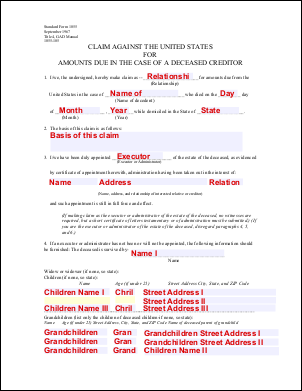eForm Placeholder ExampleThis example shows how to use eForm fields as placeholders for the insertion of text. In this example we simply replace each of the fields in a form with the name of that field.Src

 First we create an ABCpdf Doc object and read in our template form.   ```using (Doc doc = new Doc()) {   doc.Read(Server.MapPath("../mypics/form.pdf"));   doc.Form.NeedAppearances = false; // for PDF 2.0   doc.Font = doc.AddFont("Helvetica-Bold");   doc.FontSize = 16;   doc.Rect.Pin = XRect.Corner.TopLeft; ``` ```Using doc As New Doc()   doc.Read(Server.MapPath("../mypics/form.pdf"))   doc.Form.NeedAppearances = False ' for PDF 2.0   doc.Font = doc.AddFont("Helvetica-Bold")   doc.FontSize = 16   doc.Rect.Pin = XRect.Corner.TopLeft ```We iterate through each of the fields. For each field we focus on the field. We then color the rectangle light gray and draw the name of the field in dark red.   ```  string[] theNames = doc.Form.GetFieldNames();   foreach (string theName in theNames) {     Field theField = doc.Form[theName];     theField.Focus();     doc.Color.String = "240 240 255";     doc.FillRect();     doc.Rect.Height = 16;     doc.Color.String = "220 0 0";     doc.AddText(theField.Name);     doc.Delete(theField.ID);   } ``` ```  Dim theNames As String() = doc.Form.GetFieldNames()   For Each theName As String In theNames     Dim theField As Field = doc.Form(theName)     theField.Focus()     doc.Color.String = "240 240 255"     doc.FillRect()     doc.Rect.Height = 16     doc.Color.String = "220 0 0"     doc.AddText(theField.Name)     doc.Delete(theField.ID)   Next ```Finally we save.   ```  doc.Save(Server.MapPath("eform.pdf")); } ``` ```  doc.Save(Server.MapPath("eform.pdf")) End Using ```Given the following document.form.pdf This is the kind of output you might expect.eform.pdf